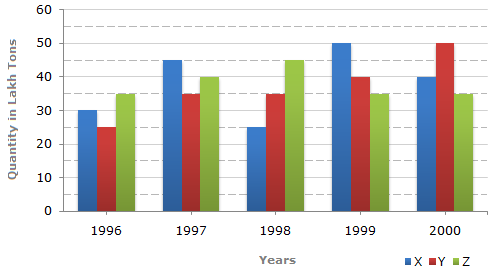# Data Interpretation - Bar Charts - Discussion

The bar graph given below shows the data of the production of paper (in lakh tonnes) by three different companies X, Y and Z over the years.

Production of Paper (in lakh tonnes) by Three Companies X, Y and Z over the Years.4.

In which year was the percentage of production of Company Z to the production of Company Y the maximum?

 [A]. 1996 [B]. 1997 [C]. 1998 [D]. 1999

Explanation:

The percentages of production of Company Z to the production of Company Z for various years are:

 For 1996 =35 x 100% = 140%. 25

 For 1997 =40 x 100% = 114.29%. 35

 For 1998 =45 x 100% = 128.57%. 35

 For 1999 =35 x 100% = 87.5%. 40

 For 2000 =35 x 100% = 70%. 50

Clearly, this percentage is highest for 1996.

 Venu said: (Jan 8, 2015) When ever we see this type of problems we can do like this. Just observe above graph no need to calculate last two years because those are negative sign. 35-25/35*100 = 28.57%. Note: Follow this formula (initial value-second value)/initial value*100.

 Ashwini said: (Nov 21, 2017) Here, the Formula is: (final value-initial value) /initial value * 100.